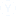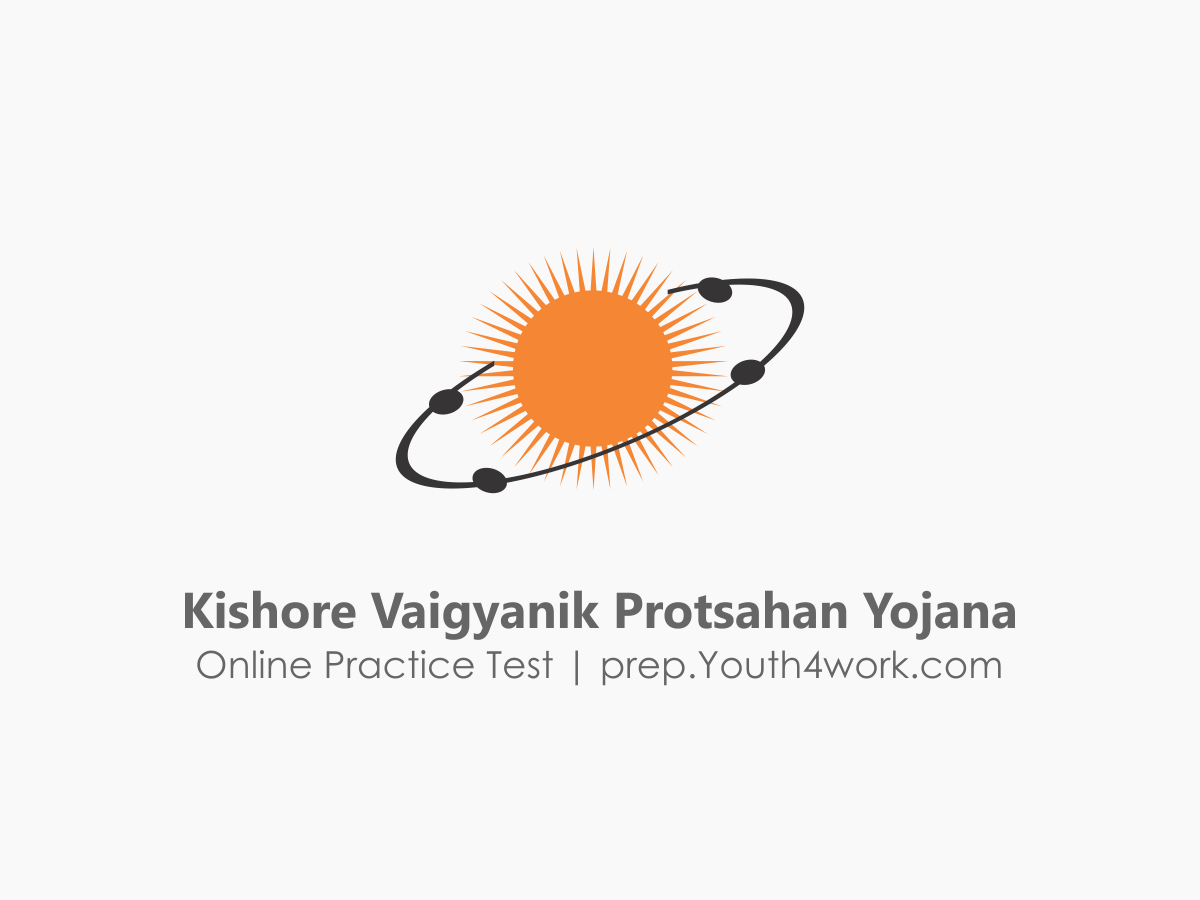# sir what is the syllabus for maths sa stream

sir the question seem to be random and I have not been able to shortlist the cho so pls help me

The Syllabus of Mathematics of KVPY 2018 is as follows:-
Mathematics
Real Number
Polynomials
Geometry
Introduction to Trigonometry
Statistics
Surface Areas and Volumes
Coordinate Geometry
Statistics and Probability
Probability

The Total duration to attempt the questions from each section is only 3 hours.Other Related Discussions